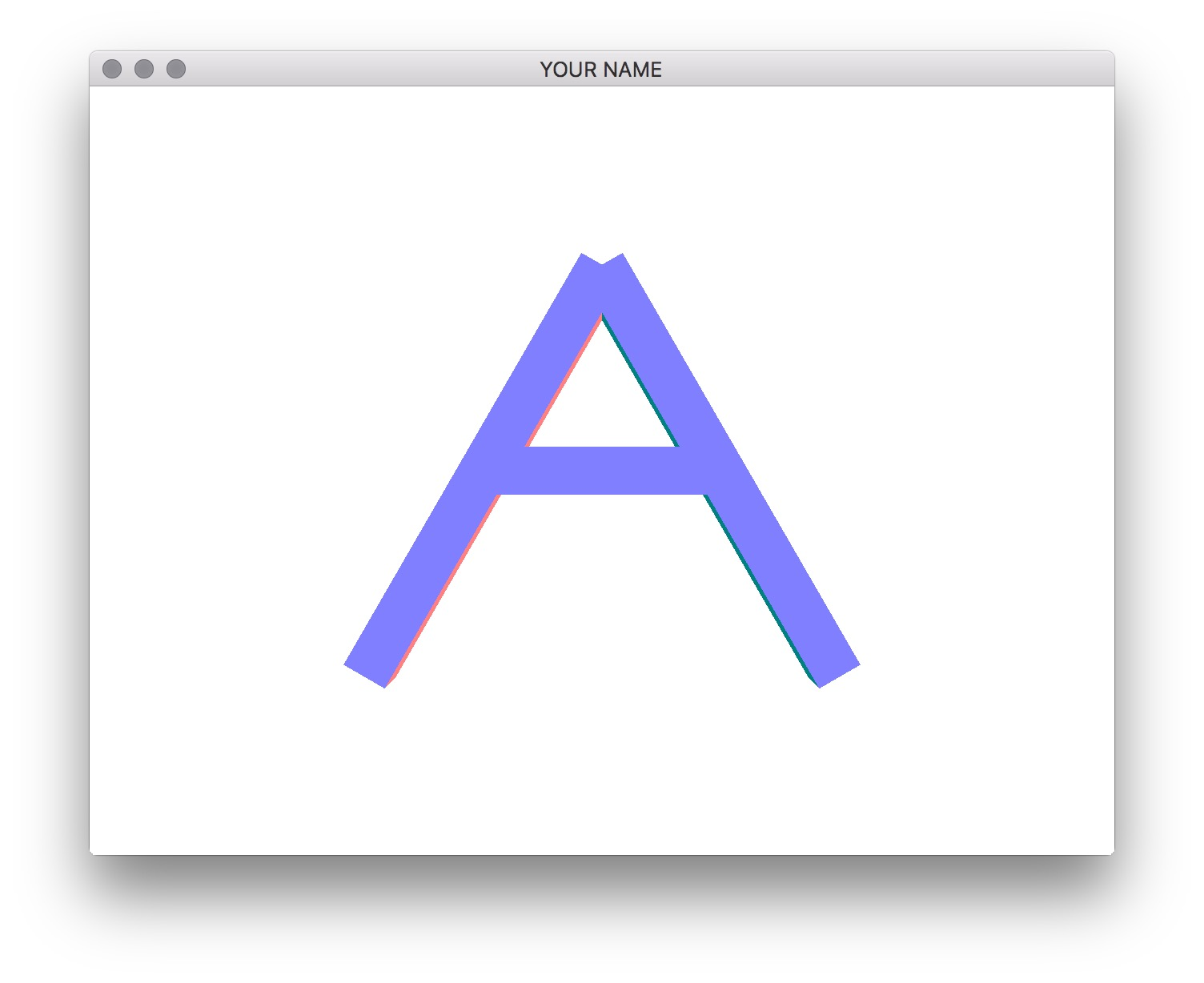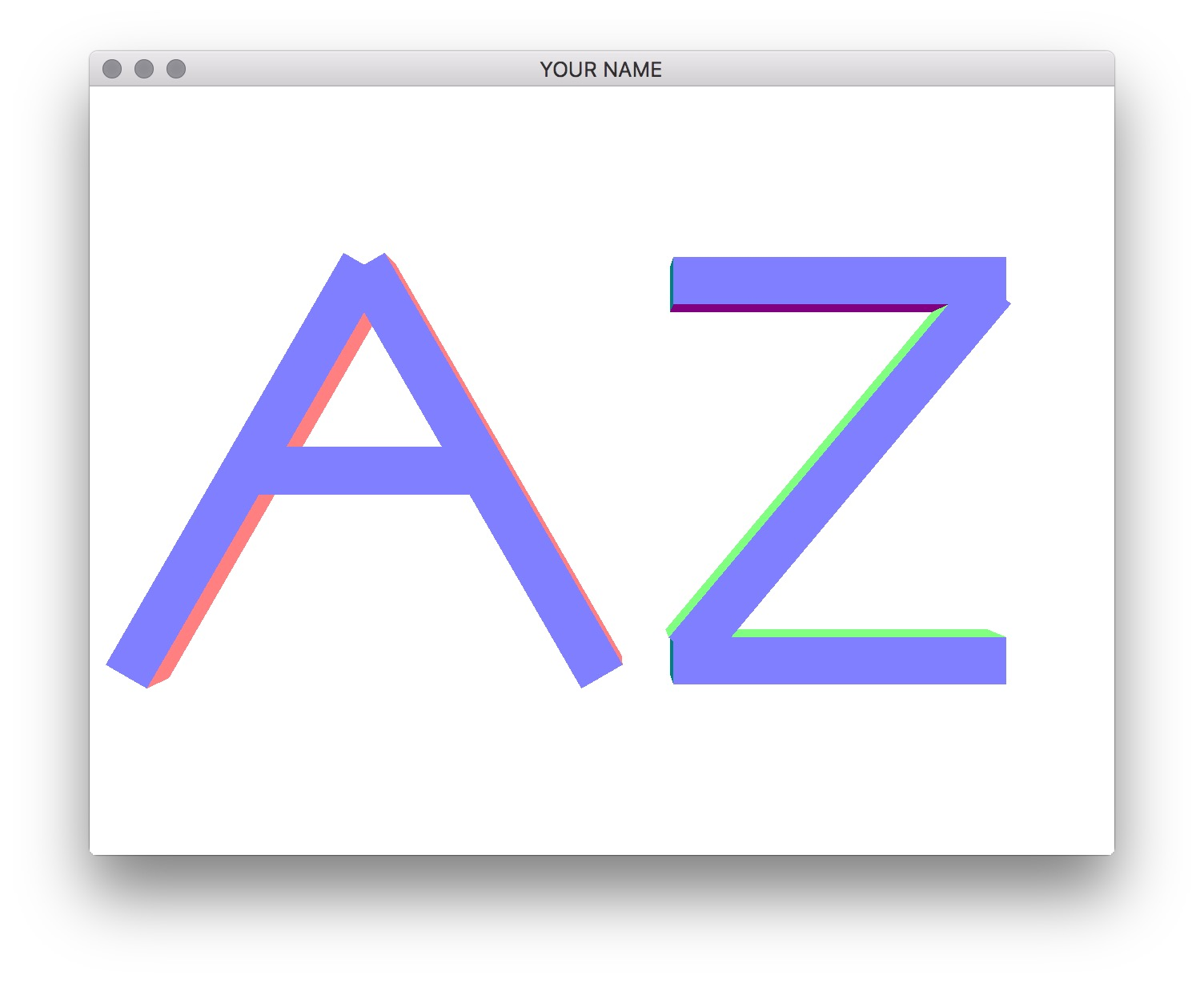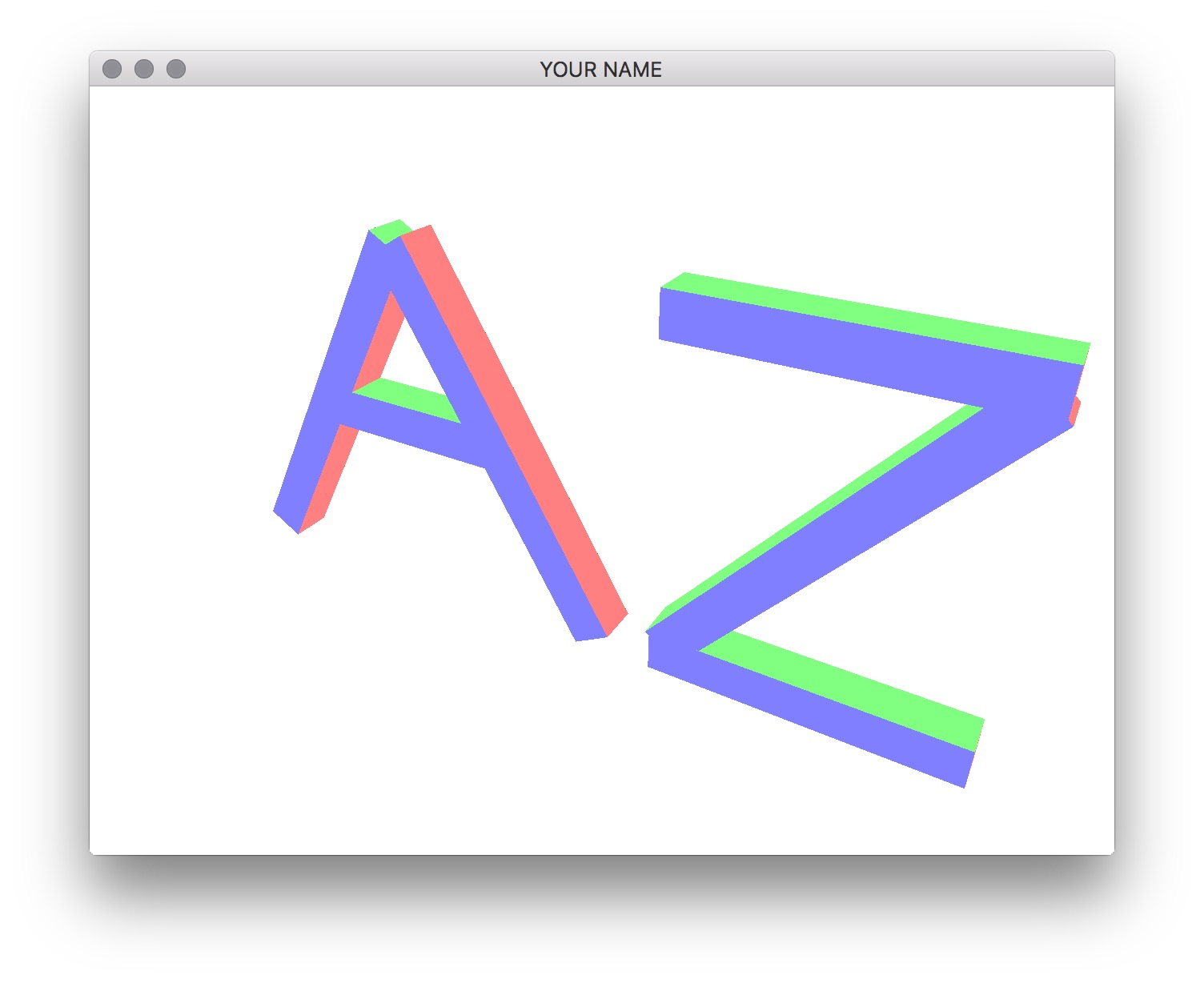# Lab 4 - Matrix Stack

## Goals for This Lab

In this lab, we will do pretty much the same thing as last time but with a matrix stack and GLM. Remember how much of a pain it was to set up the scene correctly in the last lab? You’ll see how the matrix stack makes setting up the scene a whole lot easier. With the matrix stack, it becomes much easier to reuse transformations between related objects. For example, once we create the letter A, we will now be able to translate, rotate, and scale the letter as a whole with a few simple lines of matrix stack code.

Replicate the letter A from the last lab, this time using the `MatrixStack` class. All of the functions you wrote in the last lab (plus more!) are already implemented in the `MatrixStack` class. Make sure you use `pushMatrix()` and `popMatrix()` to reuse transforms appropriately. For example, to apply a translation to all three cuboids that make up the letter A, you can do something like this:

``````MV.pushMatrix();
| MV.translate(...); // Global translation applied to all three cuboids
| MV.pushMatrix();
| | TRANSFORM AND DRAW CUBOID #1
| MV.popMatrix(); // This line undoes the transformation applied to #1
| MV.pushMatrix();
| | TRANSFORM AND DRAW CUBOID #2
| MV.popMatrix(); // This line undoes the transformation applied to #2
| MV.pushMatrix();
| | TRANSFORM AND DRAW CUBOID #3
| MV.popMatrix(); // This line undoes the transformation applied to #3
MV.popMatrix(); // This line undoes the global transformation from the 3rd line``````

To send the matrix at the top of the stack to the GPU, you can use the `glm::value_ptr(...)` function, like this:

``glUniformMatrix4fv(unifIDs["MV"], 1, GL_FALSE, value_ptr(MV.topMatrix()));``

The rotation functions take in an angle and an axis. To create a rotation matrix about the x-axis, pass in `(1,0,0)` as the axis. The MatrixStack code uses radians unlike old OpenGL functions.

The final Z translation, which pushes the object away from the camera at the origin, should be applied at the global level. In other words, it should be the first matrix stack call.

The result should be the “straight-on” A from the last lab.Move the letter A to the left. Now create the letter Z to the right using three transformed cubes again.